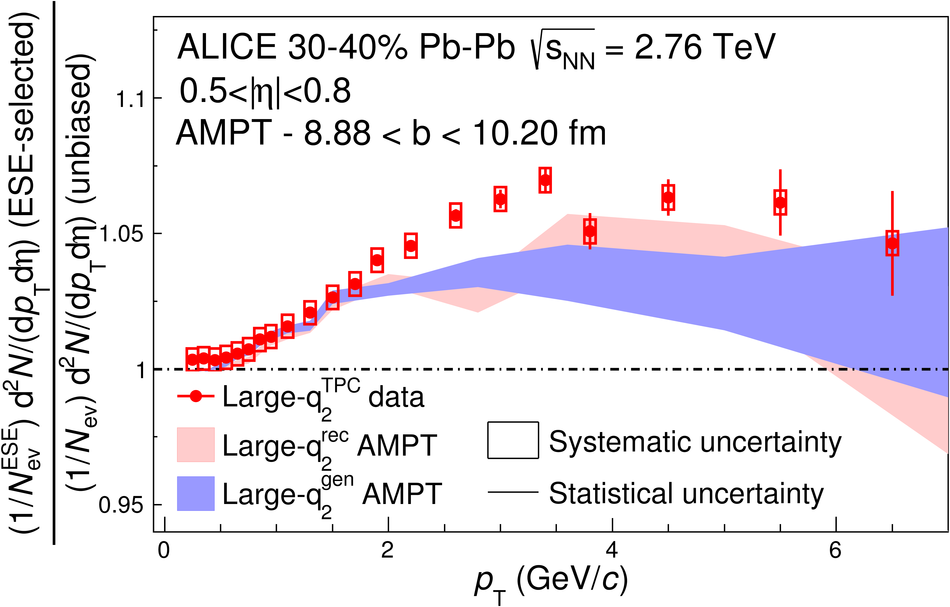# Event shape engineering for inclusive spectra and elliptic flow in Pb-Pb collisions at $\sqrt{s_\rm{NN}}=2.76$ TeV

We report on results obtained with the Event Shape Engineering technique applied to Pb-Pb collisions at $\sqrt{s_\rm{NN}}=2.76$ TeV. By selecting events in the same centrality interval, but with very different average flow, different initial state conditions can be studied. We find the effect of the event-shape selection on the elliptic flow coefficient $v_2$ to be almost independent of transverse momentum $p_\rm{T}$, as expected if this effect is due to fluctuations in the initial geometry of the system. Charged hadron, pion, kaon, and proton transverse momentum distributions are found to be harder in events with higher-than-average elliptic flow, indicating an interplay between radial and elliptic flow.

Figures

## Figure 1

 Distributions of $q_2^{\rm TPC}$ (top) and $q_2^{\rm V0C}$ (bottom) as a function of centrality (left) and projections for two centrality classes, 0-1% and 30-31% (right). In each of the left panels the solid curve shows the average $q_2$ as a function of centrality, while the dashed and the dotted curves indicate the top 10% and the bottom 10%, respectively.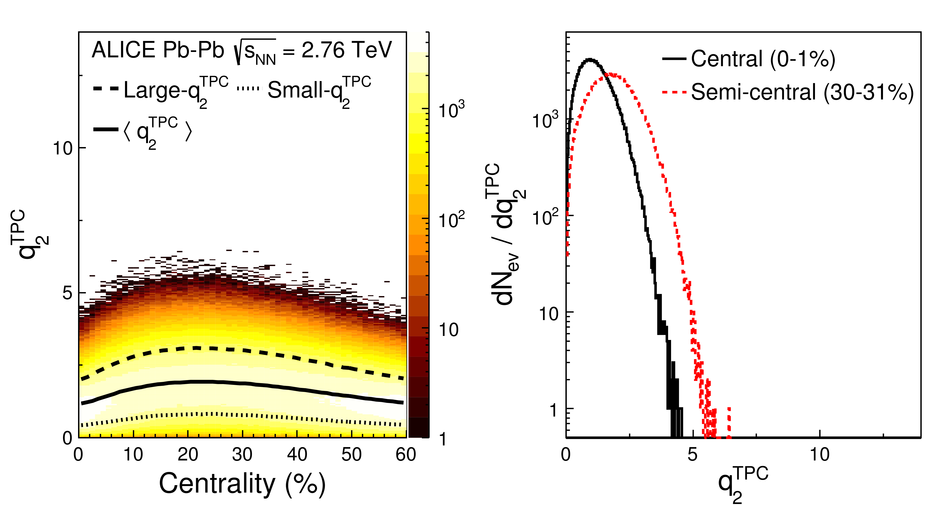## Figure 2

 Effect of the $q_2^{\rm V0C}$ ($q_2^{\rm TPC}$) event shape selection on the $q_2^{\rm TPC}$ ($q_2^{\rm V0C}$) distributions for events in the 30-31% centrality class.## Figure 3

 Measurement of $v_2\{\rm SP\}$ as a function of $\pt$ in different centrality classes for the unbiased, the large-$q_2$ and the small-$q_2$ samples. Only statistical uncertainties are plotted (systematic uncertainties are smaller than the markers).## Figure 4

 Ratio of $v_2\{\rm SP\}$ in the large-$q_2$ and small-$q_2$ samples to unbiased sample. Only statistical uncertainties are plotted (systematic uncertainties are smaller than the markers).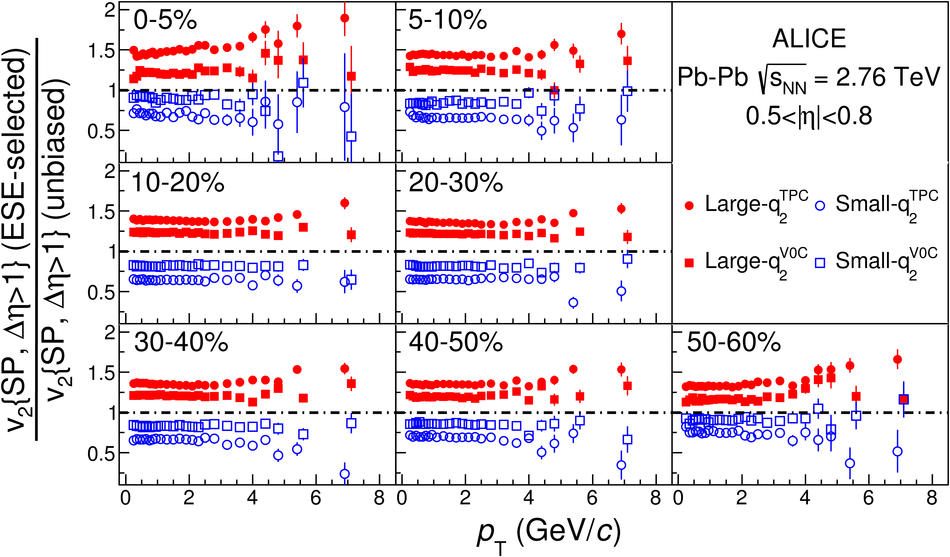## Figure 5

 Comparison between the effect of the event-shape selection obtained with the standard V0C and with the tuned TPC selections (see text for details), in the centrality class 30-40%. Top: $v_2\{\rm SP\}$, bottom: ratios to the unbiased sample. Only statistical uncertainties are plotted (systematic uncertainties are smaller than the markers).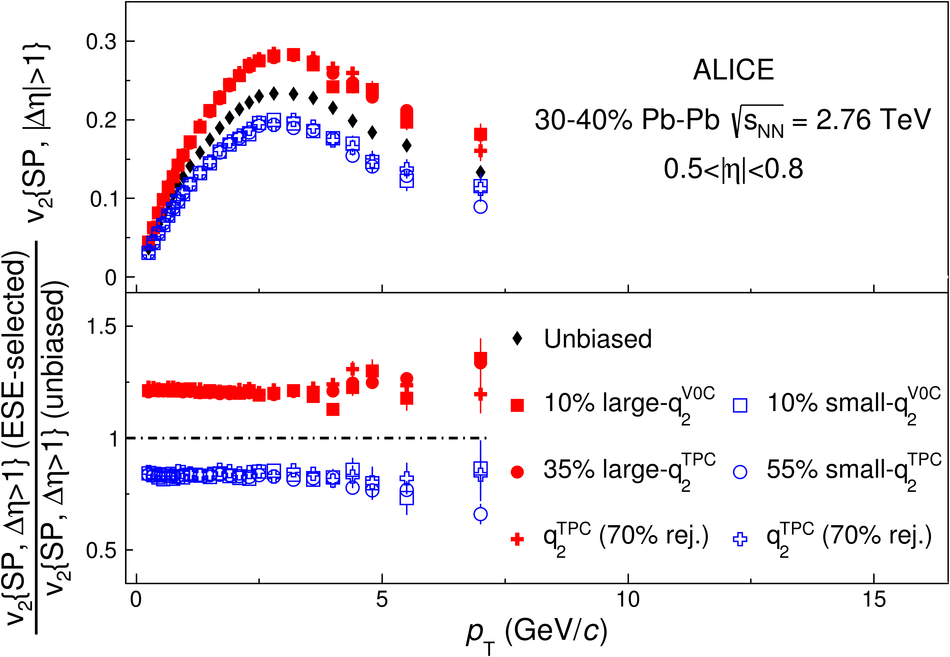## Figure 6

 Centrality dependence of the average $q_2^{\rm V0C}$ ($q_2^{\rm TPC}$ variation in the large-$q_2$ and small-$q_2$ samples.## Figure 7

 Average $v_2\{\rm SP\}$ variation as a function of the absolute (left) values and self-normalized (right) values of the $q_2^{\rm TPC}$ and $q_2^{\rm V0C}$ for several centrality classe## Figure 8

 Ratio of the $\pt$ distribution of charged hadrons in the large-$q_2$ or small-$q_2$ sample to the unbiased sample ($q_2^{\rm V0C}$ and $q_2^{\rm TPC}$ selections) in different centrality classes. Only statistical uncertainties are plotted (systematic uncertainties are smaller than the markers).## Figure 9

 Ratio of the $\pt$ distribution of charged hadrons in the large-$q_2$ or small-$q_2$ sample to the unbiased sample. Comparison between the effect of the event-shape selection obtained with the standard V0C and with the tuned TPC selections (see text for details), in the centrality class 30-40%.## Figure 10

 Ratio of the $\pt$ distribution of identified charged hadrons in the large-$q_2$ sample to the unbiased sample for the $q_2^{\rm TPC}$ (top) and $q_2^{\rm V0C}$ (bottom) selections.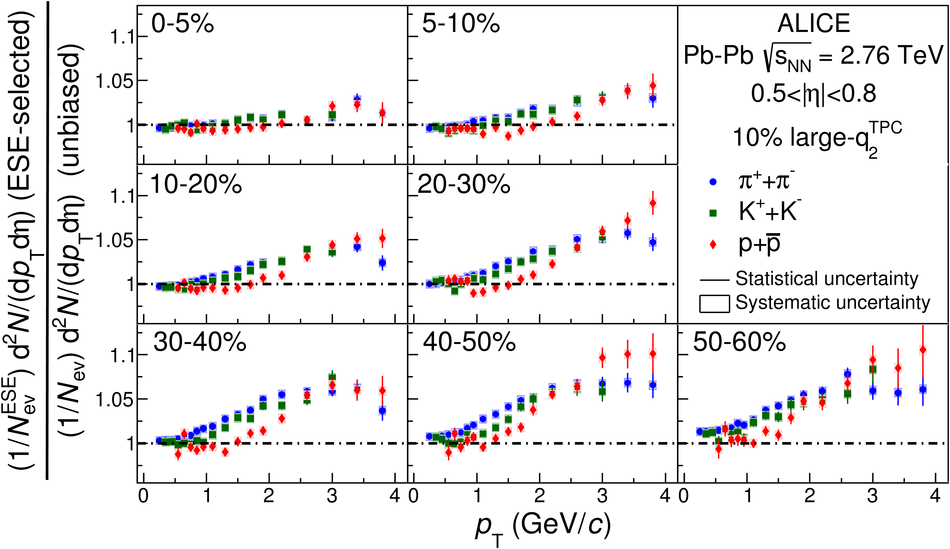## Figure 11

 Ratio of the $\pt$ distribution of identified charged hadrons in the small-$q_2$ sample to the unbiased sample for the $q_2^{\rm TPC}$ (top) and $q_2^{\rm V0C}$ (bottom) selection.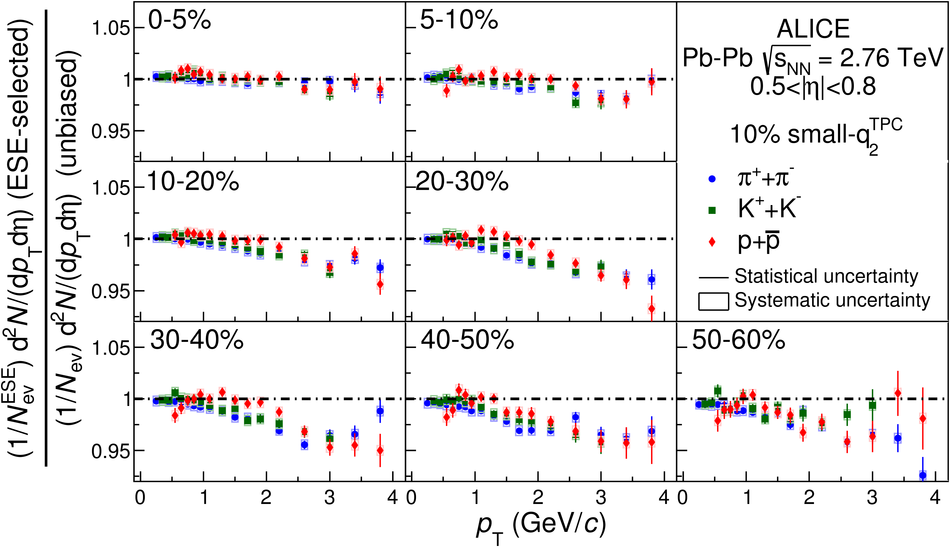## Figure 12

 Ratio of the $\pt$ distribution of identified charged hadrons in the large-$q_2$ (top) and small-$q_2$ (bottom) sample to the unbiased sample ($q_2^{\rm TPC}$ selection), in 30-40% centrality class. Lines: ratio of the blast-wave parametrizations (see text for details).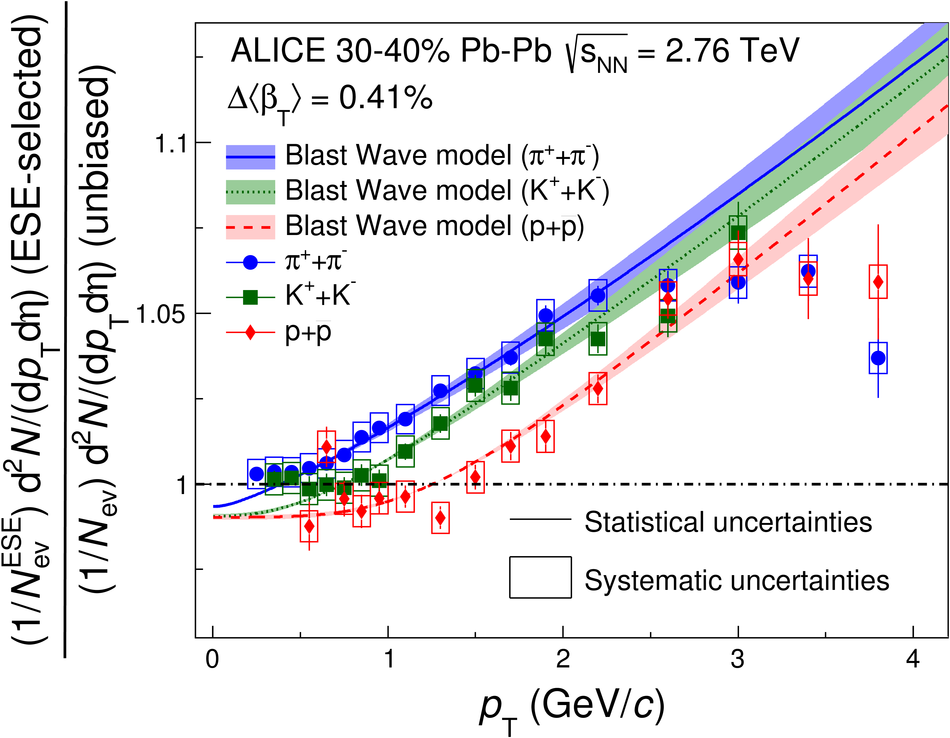## Figure 13

 Participant density as a function of the participant eccentricity estimated in a Glauber Monte Carlo model for central (top) and semi-central (bottom) collisions.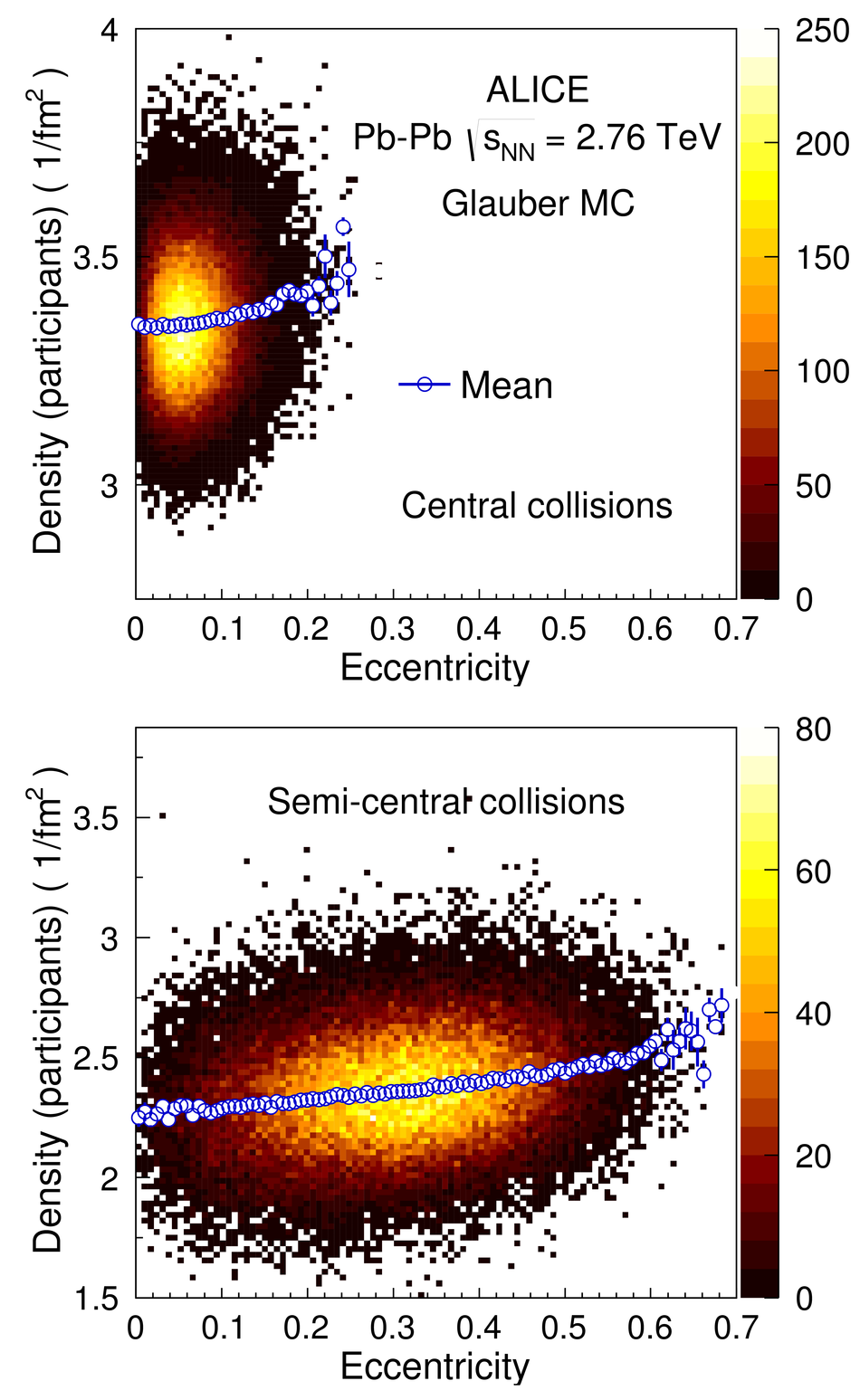## Figure 14

 Measurement of $v_2\{\rm SP\}$ as a function of $\pt$, for the unbiased sample and for the large-$q_2$ sample (top panel) and ratio between the large-$q_2$ result over the unbiased result (bottom panel). Data points (full markers) are compared with AMPT Monte Carlo model (bands). Only statistical uncertainties are plotted (systematic uncertainties are smaller than the markers).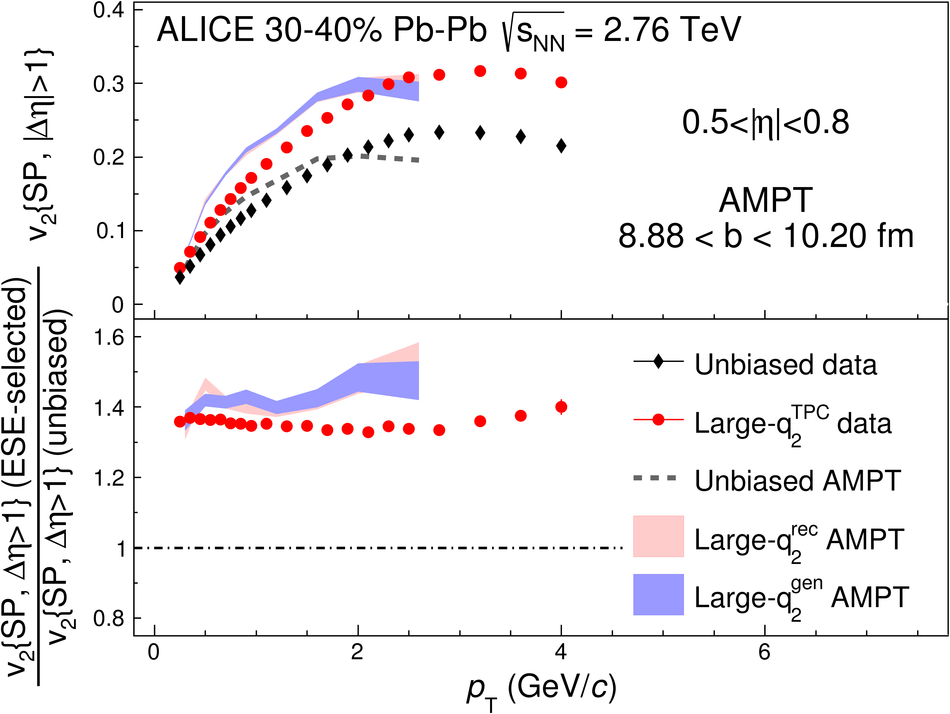## Figure 15

 Ratio of the $\pt$ distribution of charged hadrons in the large-$q_2$ sample to the unbiased sample for the $q_2^{\rm TPC}$ selection. Data points (full markers) are compared with AMPT Monte Carlo model (bands).